# 用DAX强化图表交互力

## 目标

1.可以让图表根据用户的选择，显示对应的字段的数据（跨列筛选） 2.让用户可以自由选择需要显示或需要移除的字段（以移动平均度量值为例） 3.允许用户通过传参改变图表数据背后的计算公式（以改变移动平均项数为例）

## 过程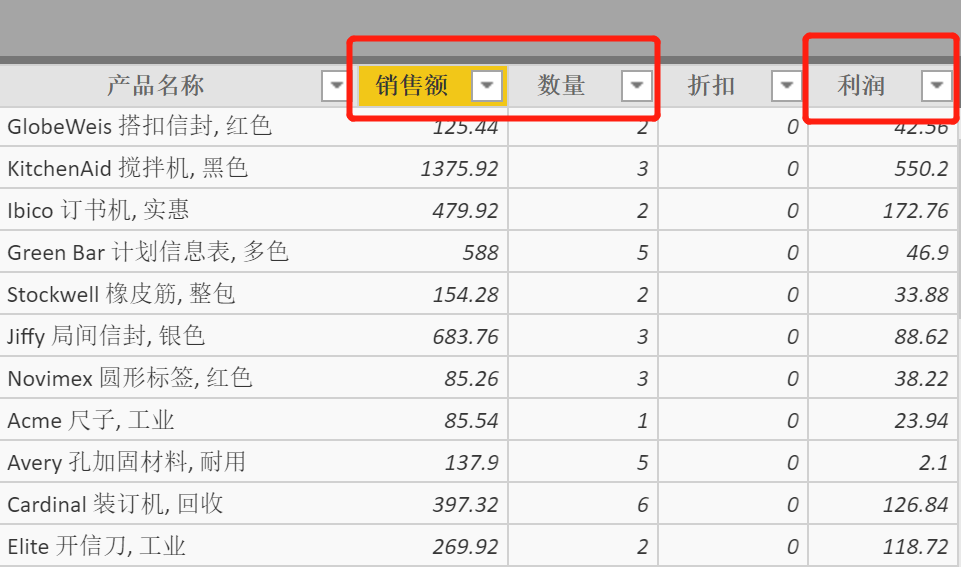也许会有一个疑问,DAX在执行计算时，基于筛选上下文和行上下文，而没有“列上下文”，怎么跨列筛选呢？实际上，你会发现如果把这个所谓的列上下文用行上下文的形式表现出来就可以解决问题了，如下：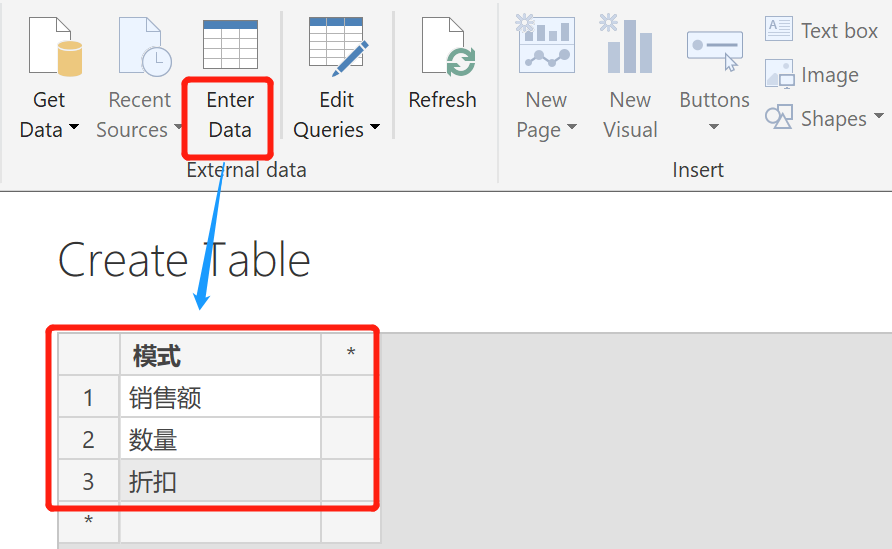``````Measure =
VAR
SELECTED = IF(HASONEVALUE('模式'[模式]),VALUES('模式'[模式]),0)
VAR
AMT = sum(Data[销售额])
VAR
QTY = SUM(Data[数量])
VAR
RETURN
SWITCH(SELECTED,"销售额",AMT,"销售量",QTY,"利润",PROFIT)
``````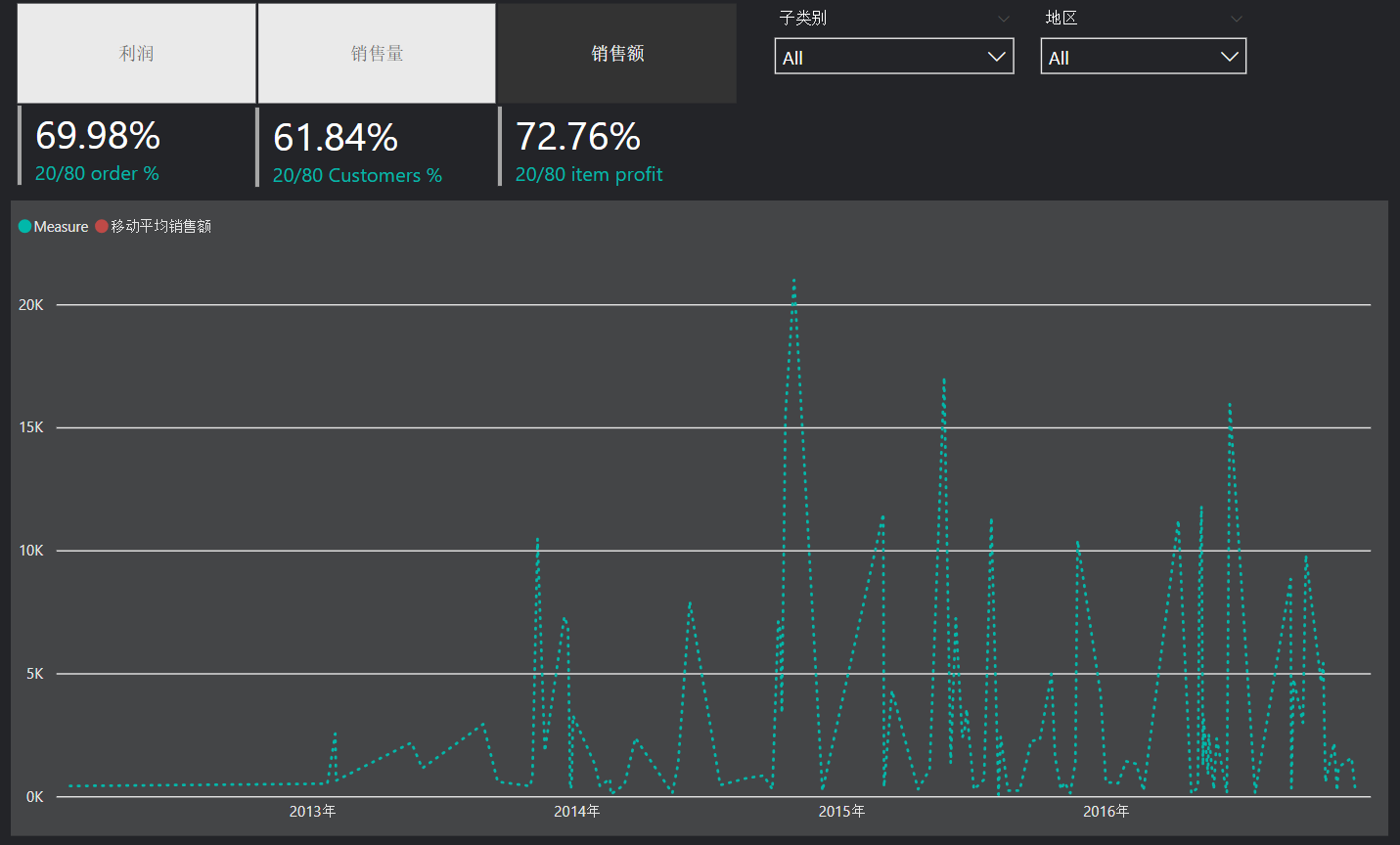• 可以让图表根据用户的选择，显示对应的字段的数据（跨列筛选）
• 让用户可以自由选择需要显示或需要移除的字段
• 允许用户通过传参改变图表数据背后的计算公式

``````移动平均销售额 =
VAR
X = 3
RETURN
CALCULATE(
AVERAGEX(
'Data',
CALCULATE(sum(Data[销售额]),
ALLEXCEPT('Data','Data'[订单日期]))),
DATESINPERIOD('Data'[订单日期],LASTDATE('Data'[订单日期]),X,MONTH))
``````

``````移动平均销售额 =
VAR
SELECTED = IF(ISFILTERED('显示&隐藏'[显示&隐藏]),VALUES('显示&隐藏'[显示&隐藏]),"F")
VAR
X = 3
VAR
MOV_AVG =
CALCULATE(
AVERAGEX(
'Data',
CALCULATE(sum(Data[销售额]),
ALLEXCEPT('Data','Data'[订单日期]))),
DATESINPERIOD('Data'[订单日期],LASTDATE('Data'[订单日期]),X,MONTH))
RETURN
SWITCH(SELECTED,"显示移动平均",MOV_AVG,"F",BLANK())
``````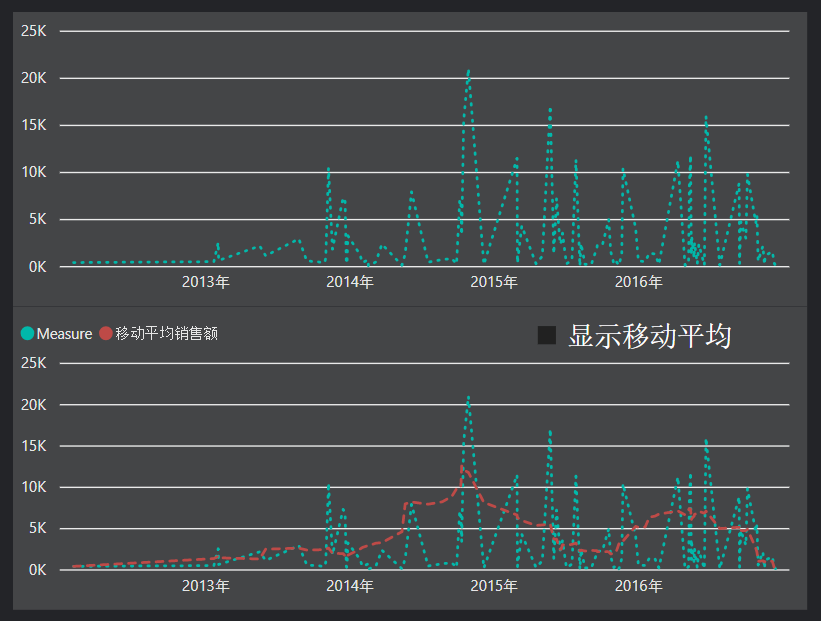• 可以让图表根据用户的选择，显示对应的字段的数据（跨列筛选）
• 让用户可以自由选择需要显示或需要移除的字段
• 允许用户通过传参改变图表数据背后的计算公式

1. 在Power BI Desktop点击【Modeling】–【New Parameter】，在【What-if parameter】界面填写序列名，数据类型，最小与最大值，默认值和递增量，将自动生成序列表和序列度量值
2. 使用GENERATESERIES()函数新建序列表，然后使用SELECTEDVALUE()函数新建序列度量值

``````移动平均销售额 =
VAR
SELECTED = IF(ISFILTERED('显示&隐藏'[显示&隐藏]),VALUES('显示&隐藏'[显示&隐藏]),"F")
VAR
X = '移动项数'[移动项数 Value]
VAR
MOV_AVG =
CALCULATE(
AVERAGEX(
'Data',
CALCULATE(sum(Data[销售额]),
ALLEXCEPT('Data','Data'[订单日期]))),
DATESINPERIOD('Data'[订单日期],LASTDATE('Data'[订单日期]),X,MONTH))
RETURN
SWITCH(SELECTED,"显示移动平均",MOV_AVG,"F",BLANK())
``````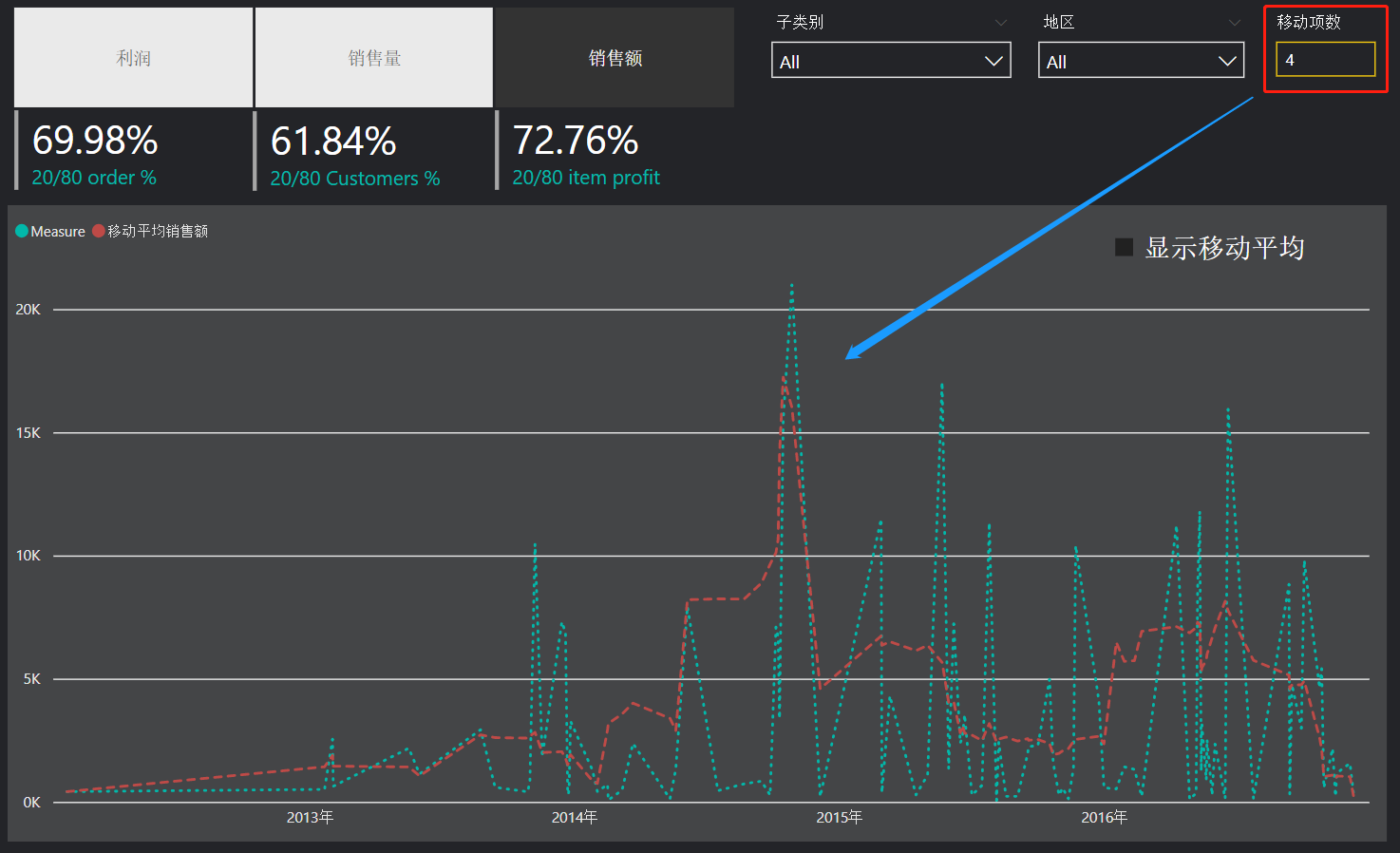• 可以让图表根据用户的选择，显示对应的字段的数据（跨列筛选）
• 让用户可以自由选择需要显示或需要移除的字段
• 允许用户通过传参改变图表数据背后的计算公式

## 其他